# Running a simple simulation model with XLSTAT

2017-10-20

This tutorial will help you set up and run a simple simulation model in Excel using the XLSTAT statistical software.

## Simulation models

Simulation models allow to obtain information, such as mean or median or confidence intervals, on variables that do not have an exact value, but for which we either know or assume a distribution. If some “result” variables depend on these “distributed” variables by the way of known or assumed formulae, then the “result” variables will also have a distribution. Sim allows you to define the distributions, and then to obtain, through simulations, an empirical distribution of the input and output variables as well as the corresponding statistics.

Simulation models are used in many areas such as finance and insurance, medicine, oil and gas prospecting, accounting, or sales prediction.

Four elements are involved in the construction of a simulation model:

1. Distributions are associated to random variables. XLSTAT gives a choice of more than 30 distributions to describe the uncertainty on the values that a variable can take. For example, you can choose a triangular distribution if you have a quantity for which you know it can vary between two bounds, but with a value that is more likely (a mode). At each iteration of the computation of the simulation model, a random draw is performed for each distribution that has been defined.
2. Scenario variables allow to include in the simulation model a quantity that is fixed in the model, except during the tornado analysis where it can vary between two bounds.
3. Result variables correspond to outputs of the model. They depend either directly or indirectly, through one or more Excel formulae, on the random variables to which distributions have been associated and, if available, on the scenario variables. The goal of computing the simulation model is to obtain the distribution of the result variables.
4. Statistics allow to track a given statistic for a result variable. For example, we might want to monitor the standard deviation of a result variable.

A correct model should comprise at least one distribution and one result variable. Models can contain any number of these four elements. A model can be limited to a single Excel sheet or can use a whole Excel folder.

## Dataset for creating and running a simple simulation model

An Excel sheet containing both the data and the results for use in this tutorial can be downloaded by clicking here.

In this tutorial, a very simple simulation model is built using two distributions and one result in order to explain the basics of simulation modeling. Further tutorials with all of the 4 model elements and options can be found under the following links: scenario variables, distribution simulation, and correlations.

Our simulation model is based on sales and costs of a shop. The benefit is simply the difference between sales and costs in this simple case. Based on historical data for costs and sales that were analyzed with the tool “distribution fitting” we found out that the costs follow a normal distribution (mu=120, sigma=10) and the sales a normal distribution (mu=80, sigma=20) (see tutorial on distribution fitting for more details).

Based on this model, the different model variables are created: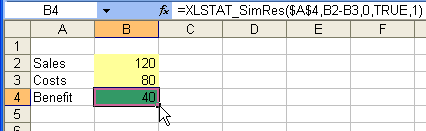This model can be found in the sheet Model.

## Creating a simple simulation model

### Creating the first distribution variable

To create the first distribution variable, select the cell B2, which corresponds to the amount of sales.

Once XLSTAT is activated, select the XLSTAT / Sim / Define a distribution command, or click on the corresponding button of the Sim toolbar (see below).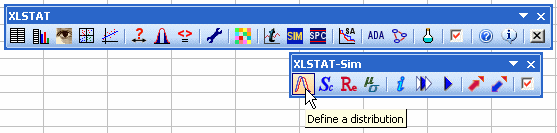The Define a distribution dialog box appears. Then select as Variable name the cell A2 with the name “Sales”. Choose a normal distribution with mu = 120 and sigma = 10.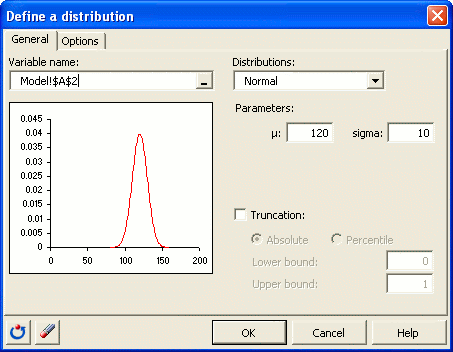Once you have clicked on OK, the corresponding function call is inserted into the active cell.

### Creating the second distribution variable

Now the second distribution variable can be generated in the same way. Select in this case a normal distribution with mu = 80 and sigma = 20. Here is the corresponding dialog box: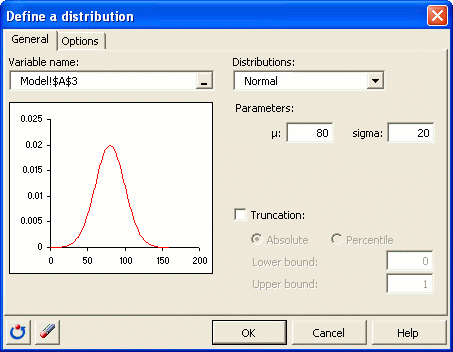### Creating the result variable

Select the result cell that contains the value 40 as result of the formula =B2-B3 as active cell. Then click the XLSTAT / Sim / Define a result variable command in the XLSTAT menu, or click on the corresponding button of the Sim toolbar.

The Define a result variable dialog box is displayed. Then select cell A4 as variable name.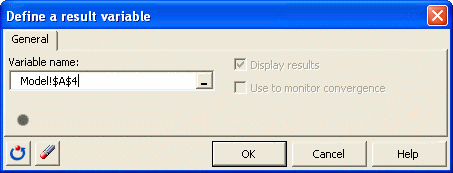Once you have clicked on OK, the corresponding function call to XLSTAT_SimRes is inserted into the active cell.

This can be found in the Excel sheet Model.

## Running a simple simulation model

To start the simulation run, select the XLSTAT / Sim / Simulation - Run command, or click on the corresponding button of the Sim toolbar.

The Simulation - run dialog is displayed. You can set the number of simulations to 1000.In the Options tab, enter the parameters of the tornado and spider analyses.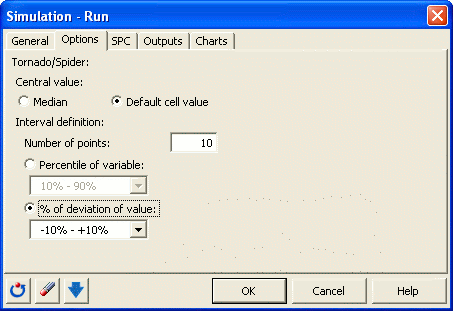The computations begin once you have clicked on OK.

## Interpreting the results of a simple simulation model

The first result is a summary of the simulation model.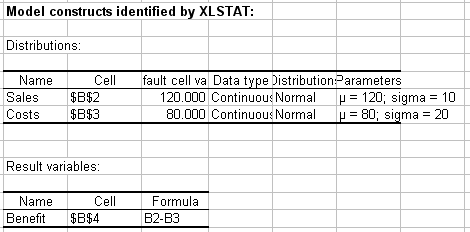Then, details on the two distribution variables and on the result variable are displayed.

The following tables show details for the two distribution variables (descriptive statistics, histograms and quantiles).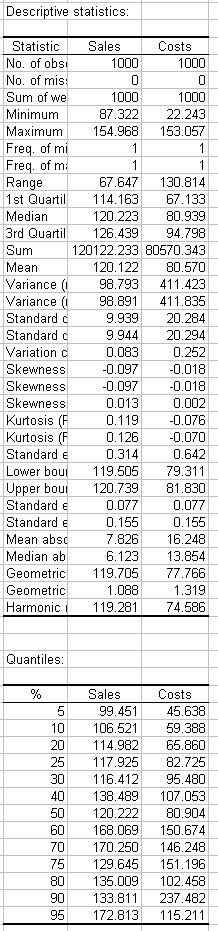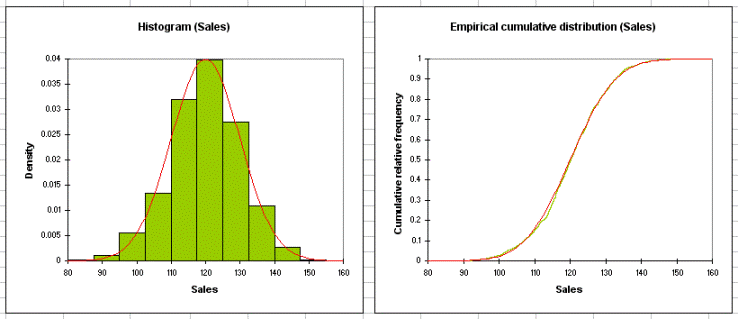The following tables show details for the result variable. Descriptive statistics, a histogram and statistics about the intervals are displayed. Then the results of the sensitivity analysis are shown. The sensitivity analysis is based on the simulations contrary to the tornado analysis presented below.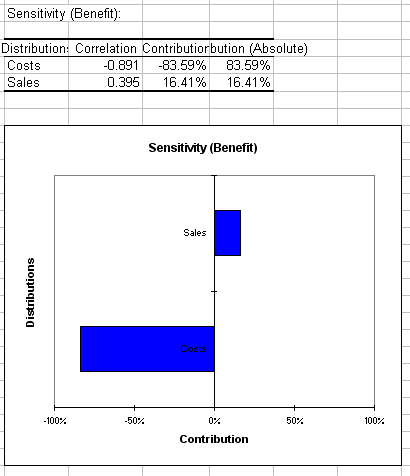The next section contains the tornado analysis.

Tornado analysis is not based on the iterations of the simulation but on a point by point analysis of all the input variables (random variables with distributions and scenario variables).

During the tornado analysis, for each result variable, each input random variable and each scenario variable are studied one by one. We make their value vary between two bounds and record the value of the result variable, in order to know how each random and scenario variable impacts the result variable. For a random variable, the values explored can either be around the median or around the default cell value, with bounds defined by percentiles or deviation. For a scenario variable, the analysis is performed between two bounds specified when defining the variables. The number of points is an option that can be modified by the user before running the simulation model.

In the diagram we see that the costs have the strongest impact on the benefit. These results are not based on the iterations of the simulation.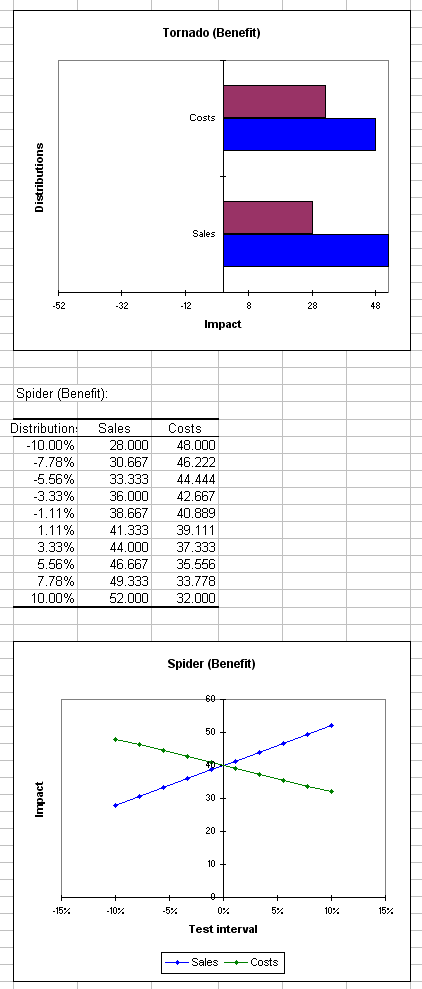Finally the correlation matrix of the distributions and result variables are displayed. We see that the costs and the sales are not correlated. But the benefit is of course correlated to sales and costs.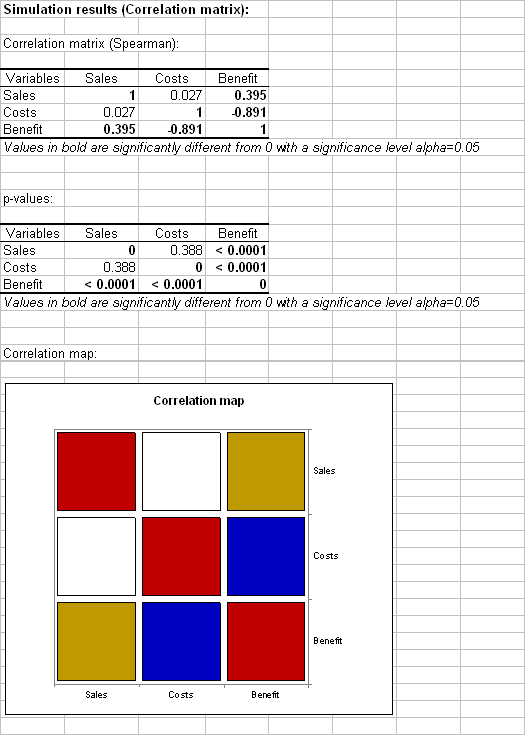#### Contact our technical support team: support@xlstat.com

https://cdn.desk.com/
false
desk